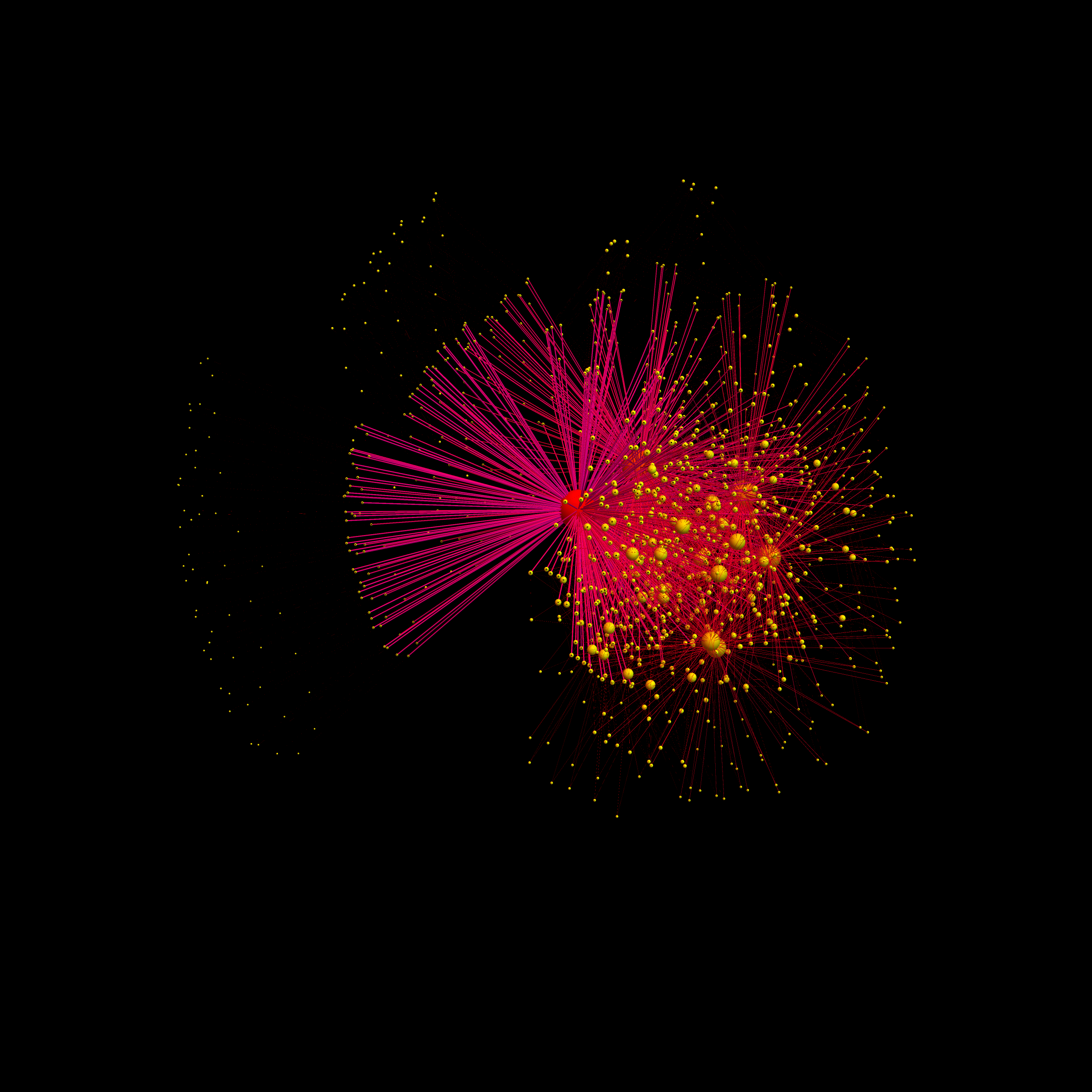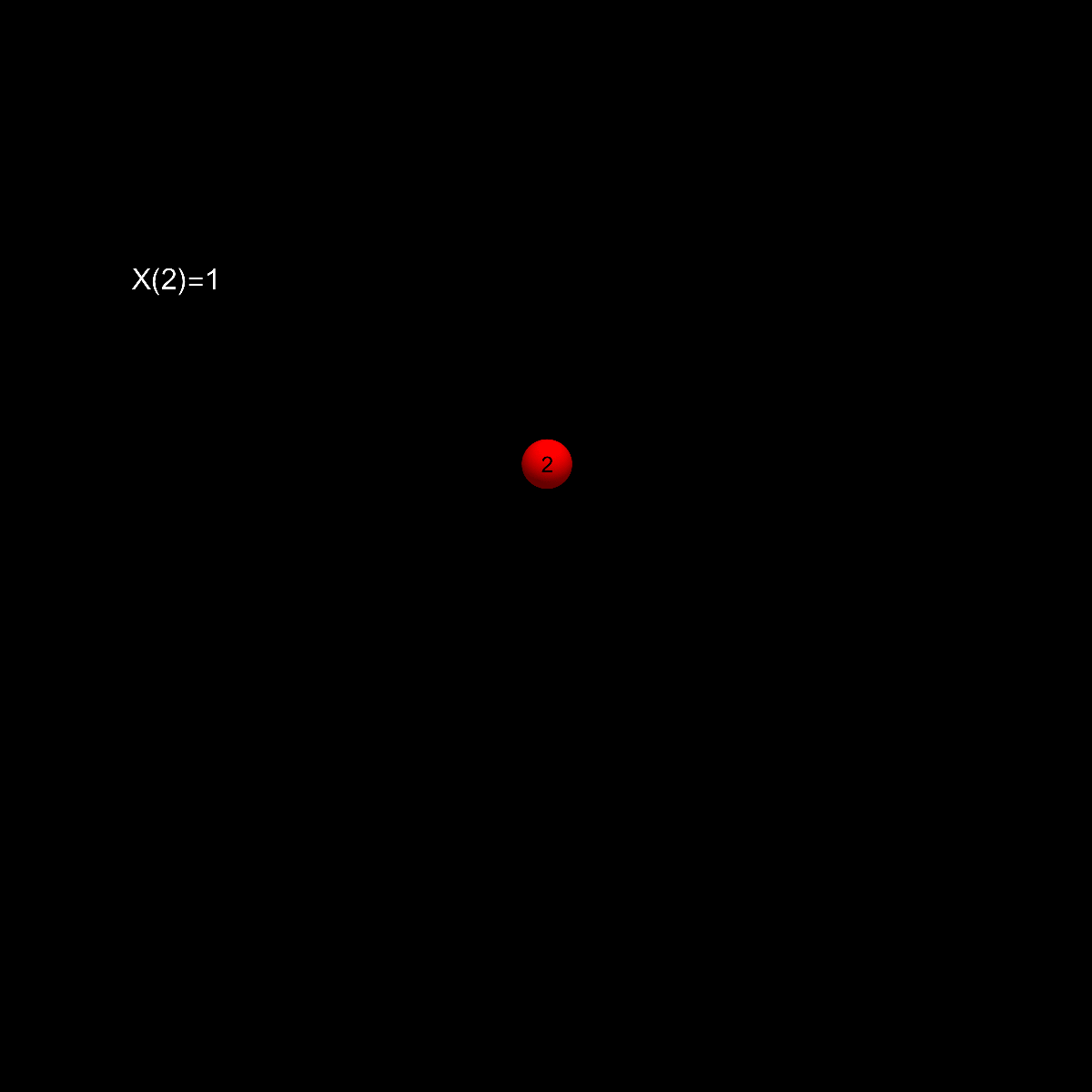• August 29: the following picture shows the connected component of the prime graph for n = 2*3*5*7*11 = 2310. The vertices are all the integers between 2 and n which are square free. Two integers are connected if one is a factor of the other: